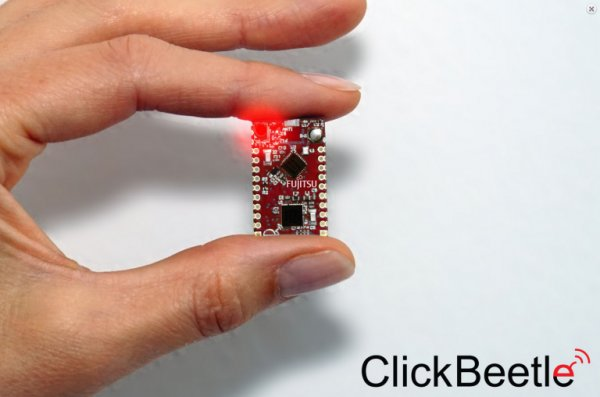How to make precision measurements on a nanopower budget, part 1: DC gain in nanopower op amps

Heightened accuracy and speed in an operational amplifier (op amp) has a direct relationship with the magnitude of its power consumption. Decreasing the current consumption decreases the gain bandwidth; conversely, decreasing the offset voltage increases the current consumption.Many such interactions between op amp electrical characteristics influence one another. With the increasing need for low power consumption in applications like wireless sensing nodes, the Internet of Things (IoT) and building automation, understanding these trade-offs has become vital to ensure optimal end-equipment performance with the lowest possible power consumption. In the first installment of this two-part blog post series, I’ll describe some of the power-to-performance trade-offs of DC gain in precision nanopower op amps.

DC gain

You probably remember from school the classic inverting (Figure 1) and noninverting (Figure 2) gain configurations of op-amps.

Using these equations, you can see that you must choose large resistor values that provide both the gain you need while minimizing power dissipation (and therefore power consumption). If you don’t minimize current through the feedback path, you’ll lose the benefit of using manpower op amps.

Once you’ve determined what resistor values will meet your gain and power-consumption needs, you’ll need to consider some of the other op amp electrical characteristics that will affect the accuracy of signal conditioning. Summing several small systemic errors inherent in nonideal op amps will give you the total offset voltage. The electrical characteristic, VOS, is defined as a finite offset-voltage number between the op amp inputs, and describes these errors at a defined bias point. Please note that it does not describe these errors across all operating conditions. To do that, you must consider the gain error, bias current, voltage noise, common-mode rejection ratio (CMRR), power-supply rejection ratio (PSRR) and drift. Covering all of these parameters is beyond the scope of this post, but let’s look at VOS and drift – and their influence in nanopower applications – in a bit more detail.

READ  Precision programmable current source uses two ICs

Real-world op-amps exhibit VOS across their input terminals, which can sometimes be a problem in low-frequency (close to DC) precision signal-conditioning applications. In voltage gain configurations, the offset voltage will gain up along with the signal being conditioned, introducing measurement errors. In addition, the magnitude of VOS can change over both time and temperature (drift). Therefore, in low-frequency applications requiring fairly high-resolution measurements, it’s important to select a precise (VOS ≤ 1mV) op-amp with the lowest possible drift.

Read More: How to make precision measurements on a nano power budget, part 1: DC gain in nano power op amps